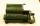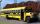Mistake

Nicol mistake when calculate in school. Instead of add number 20 subtract it.

What is the difference between the result and the right result?

Result

x =  -40

Solution:Leave us a comment of this math problem and its solution (i.e. if it is still somewhat unclear...):Be the first to comment!To solve this verbal math problem are needed these knowledge from mathematics:

Do you have a linear equation or system of equations and looking for its solution? Or do you have quadratic equation?

Next similar math problems:

1. I thinkI think a number. When I multiply it by five, and after that I subtract 477, I get the same number as if I multiplied it twice. What number do I think?
2. I think numberI think number.When I add 841 to it and subtract 157, I get a number that is 22 greater than 996. What number I thinking?
3. Roman numerals 2-Subtract up the number written in Roman numerals. Write the results as Roman numbers.
4. What is missing (1000)What number is to add to get 1000?
5. Seven timesWhich number seven times is just as higher as 27, how much is smaller than 29?
6. Roman numerals 2+Add up the number writtens in Roman numerals. Write the results as a roman numbers.
7. Addition of Roman numbersAdded together and write as decimal number: LXVII + MLXIV
8. SubtractionTest what do you know about the subtraction of two numbers: a) make a difference if the minuend 4,307,288 and subtrahend is 472008 b) minuend is 4000 more than subtrahend. What's the difference? c) the difference is 38900 less than the minuend. What is th
9. IntegersMay be the sum of two integers less than their difference?
10. SummandOne of the summands is 145. The second is 10 more. Determine the sum of the summands.
11. Write decimalsWrite in the decimal system the short and advanced form of these numbers: a) four thousand seventy-nine b) five hundred and one thousand six hundred and ten c) nine million twenty-six
12. Two numbers 6Fill two natural numbers a, b: 7 + blank- blank = 5
13. DriveAt the bus stop 8 people take out and 10 people take into bus. Next stop 6 take out and 5 take in. On the third stop 6 take out and 3 take in. The bus traveled further with 39 people. How many passengers were originally at the bus?
14. Eq1Solve equation: 4(a-3)=3(2a-5)
15. Simple equation 8Solve the following equation: 36=-(1+7x)-6(-7-x)
16. If-then equationIf 5x - 17 = -x + 7, then x =
17. Six te 2If 3t-7=5t, then 6t=